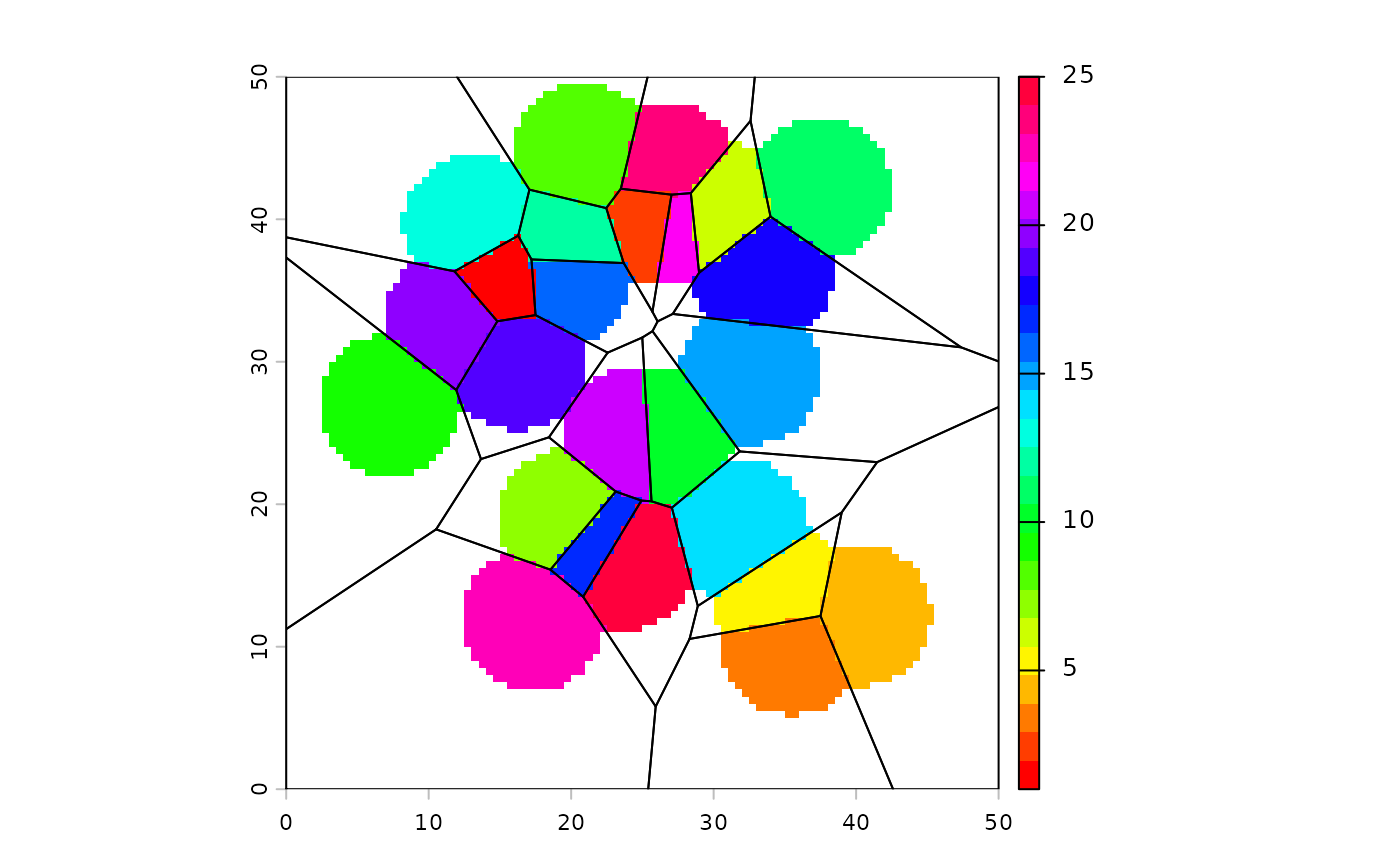Nearest neighbor interpolation of points, using a moving window

# S4 method for SpatRaster,SpatVector
interpNear(x, y, field, radius, interpolate=FALSE, fill=NA, filename="", ...)

# S4 method for SpatRaster,matrix
interpNear(x, y, radius, interpolate=FALSE, fill=NA, filename="", ...)

## Arguments

x

SpatRaster

y

SpatVector or matrix with three columns (x,y,z)

field

character. field name in SpatVector y

numeric. The radius of the circle (single number). If interpolate=FALSE it is also possible to use two or three numbers. Two numbers are interpreted as the radii of an ellipse (x and y-axis). A third number should indicated the desired, counter clockwise, rotation of the ellipse (in degrees)

interpolate

logical. Should the nearest neighbor values be linearly interpolated between points?

fill

numeric. value to use to fill empty cells

filename

character. Output filename

...

additional arguments for writing files as in writeRaster

rasterizeWin, rasterize, interpIDW, interpolate

SpatRaster

## Examples


r <- rast(ncol=100, nrow=100, crs="local", xmin=0, xmax=50, ymin=0, ymax=50)
set.seed(100)
x <- runif(25, 5, 45)
y <- runif(25, 5, 45)
z <- sample(25)
xyz <- cbind(x,y,z)